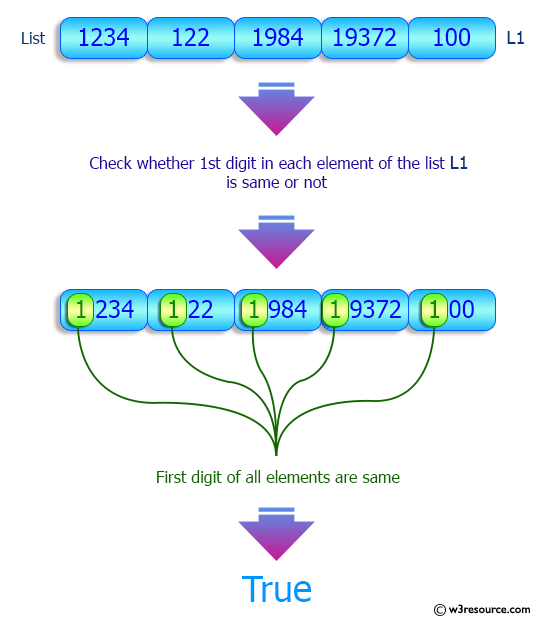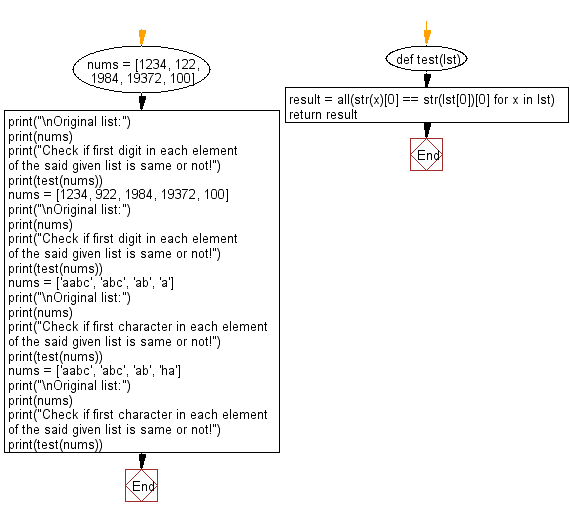﻿ Python: Check if first digit/character in each element in a given list is same or not - w3resource# Python: Check if first digit/character in each element in a given list is same or not

## Python List: Exercise - 204 with Solution

Write a Python program to check if first digit/character of each element in a given list is same or not.

Sample Solution:

Python Code:

``````def test(lst):
result = all(str(x) == str(lst) for x in lst)
return result
nums = [1234, 122, 1984, 19372, 100]
print("\nOriginal list:")
print(nums)
print("Check if first digit in each element of the said given list is same or not!")
print(test(nums))
nums = [1234, 922, 1984, 19372, 100]
print("\nOriginal list:")
print(nums)
print("Check if first digit in each element of the said given list is same or not!")
print(test(nums))
nums = ['aabc', 'abc', 'ab', 'a']
print("\nOriginal list:")
print(nums)
print("Check if first character in each element of the said given list is same or not!")
print(test(nums))
nums = ['aabc', 'abc', 'ab', 'ha']
print("\nOriginal list:")
print(nums)
print("Check if first character in each element of the said given list is same or not!")
print(test(nums))
```
```

Sample Output:

```Original list:
[1234, 122, 1984, 19372, 100]
Check if first digit in each element of the said given list is same or not!
True

Original list:
[1234, 922, 1984, 19372, 100]
Check if first digit in each element of the said given list is same or not!
False

Original list:
['aabc', 'abc', 'ab', 'a']
Check if first character in each element of the said given list is same or not!
True

Original list:
['aabc', 'abc', 'ab', 'ha']
Check if first character in each element of the said given list is same or not!
False
```

Pictorial Presentation:Flowchart:## Visualize Python code execution:

The following tool visualize what the computer is doing step-by-step as it executes the said program:

Python Code Editor:

Have another way to solve this solution? Contribute your code (and comments) through Disqus.

What is the difficulty level of this exercise?

Test your Python skills with w3resource's quiz

﻿

## Python: Tips of the Day

Floor Division:

When we speak of division we normally mean (/) float division operator, this will give a precise result in float format with decimals.

For a rounded integer result there is (//) floor division operator in Python. Floor division will only give integer results that are round numbers.

```print(1000 // 300)
print(1000 / 300)```

Output:

```3
3.3333333333333335```